## 2012年12月23日 星期日

### 觀察 ADO.NET Entity Framework 5.0 所產生的 SQL Command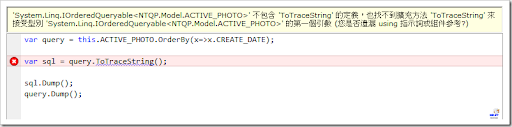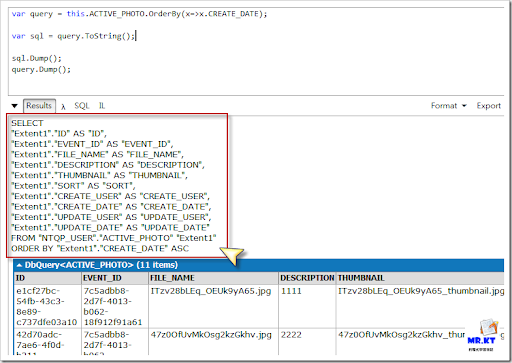http://msdn.microsoft.com/zh-tw/library/system.data.entity.infrastructure.dbquery.tostring(v=vs.103).aspx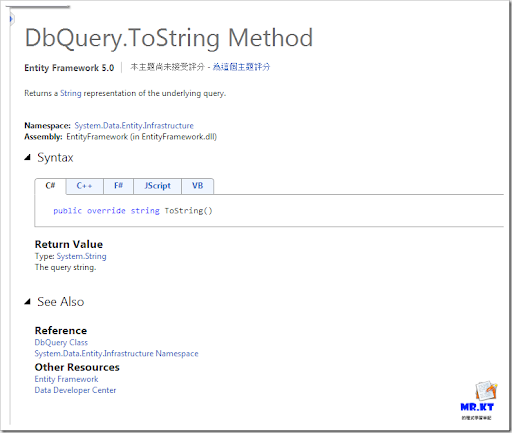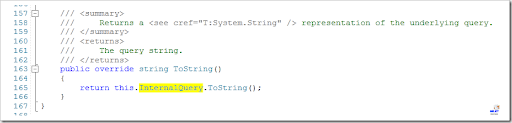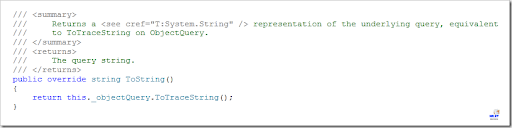### 取得 Entity 對應的 Table Name

`public class ContextExtensions`
`    {`
`        #region -- GetTableName --`
`        /// <summary>`
`        /// Gets the name of the table.`
`        /// </summary>`
`        /// <typeparam name="T"></typeparam>`
`        /// <param name="context">The context.</param>`
`        /// <param name="dbType">Type of the db.</param>`
`        /// <returns></returns>`
`        public static string GetTableName<T>(ObjectContext context, DataBaseType dbType = DataBaseType.MS_SQL_SERVER) where T : class`
`        {`
`            string sql = context.CreateObjectSet<T>().ToTraceString();`
`            string matchWords = string.Empty;`
`            switch (dbType)`
`            {`
`                case DataBaseType.MS_SQL_SERVER:`
`                    matchWords = "FROM (?<table>.*) AS";`
`                    break;`
`                case DataBaseType.Oracle:`
`                    matchWords = "FROM \"(?<schema>.*)\".\"(?<table>.*)\"\\s";`
`                    break;`
`            }`
`            Regex regex = new Regex(matchWords);`
`            Match match = regex.Match(sql);`
`            string table = match.Groups["table"].Value;`
`            return table;`
`        }`
`        #endregion`
`        public enum DataBaseType`
`        {`
`            MS_SQL_SERVER = 0,`
`            Oracle = 1`
`        }`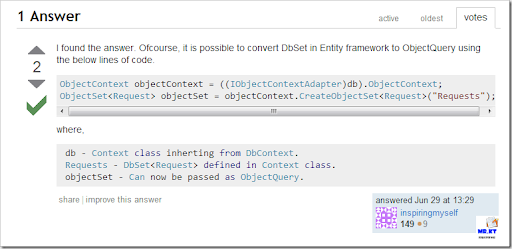http://msdn.microsoft.com/en-us/library/system.data.entity.dbcontext(v=vs.103).aspx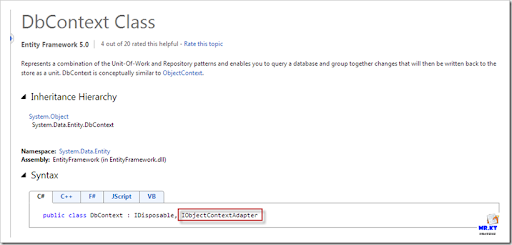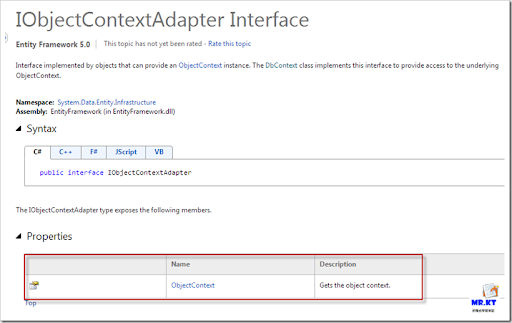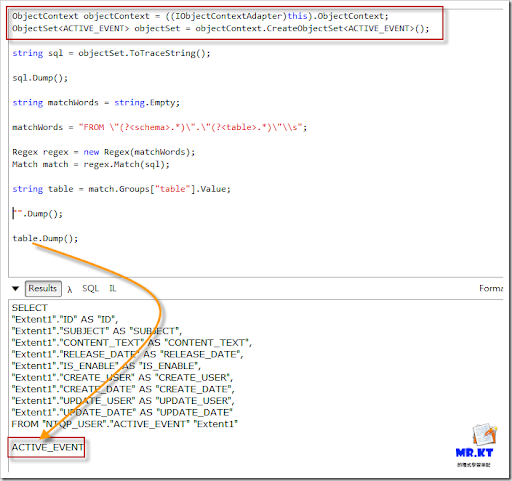`#region -- GetTableName --`
`/// <summary>`
`/// Gets the name of the table.`
`/// </summary>`
`/// <typeparam name="T"></typeparam>`
`/// <param name="context">The context.</param>`
`/// <param name="dbType">Type of the db.</param>`
`/// <returns></returns>`
`public static string GetTableName<T>(DbContext context, DataBaseType dbType = DataBaseType.Oracle) where T : class`
`{`
`    ObjectContext objectContext = ((IObjectContextAdapter)context).ObjectContext;`
`    ObjectSet<T> objectSet = objectContext.CreateObjectSet<T>();`
` `
`    string sql = objectSet.ToTraceString();`
` `
`    string matchWords = string.Empty;`
` `
`    switch (dbType)`
`    {`
`        case DataBaseType.MS_SQL_SERVER:`
`            matchWords = "FROM (?<table>.*) AS";`
`            break;`
` `
`        case DataBaseType.Oracle:`
`            matchWords = "FROM \"(?<schema>.*)\".\"(?<table>.*)\"\\s";`
`            break;`
`    }`
` `
`    Regex regex = new Regex(matchWords);`
`    Match match = regex.Match(sql);`
` `
`    string table = match.Groups["table"].Value;`
`    return table;`
`}`
`#endregion`

### LingzhiSun's Blog

Entity Framework 小技巧一 —— 如何从DbContext得到其内部封装的ObjectContext

Entity Framework 小技巧五 —— 如何得到EF查询生成的SQL？

#### 3 則留言:

1.2.請問
ToString()可以直接產生SQL command
為什麼不直接用string sql = context.Set.ToString();
然後直接Regex

1.這篇文章是五年多前所寫的，我巳經不太記得當時的情況